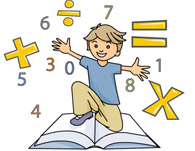Rachael Stockton

Welcome to
Mrs.Stockton's
Web Page

Math ASI

February,2020

15 minutes a day of facts practiceSTUDY BASIC FACTS EVERY DAY!

Please review basic skills each night:

multiplication/division flash cards

Use those great sites to keep your skills sharp: sum dog, study island, cool math gamesPlease feel free to e-mail me with any questions or concerns.

rstockton@hesk6.us

Homework will be posted on the homeroom teacher's site and should be completed each day.

The best way for your child to become proficient in math is to practice the basic math facts every day.

Multiplication and division facts- grades 3-6

Topics of study through the current month:It is important to remember that practice makes perfect.  Studying math facts for a minimum of 10 minutes per night will greatly improve your basic fact knowledge and lead to greater success in all areas of math.

LOOK AT WHAT WE ARE LEARNING!

• Grade 1 : Adding within 10, doubles,near doubles partners of 10, adding and subtracting in word problems, counting on to subtract, subtracting to compare, adding numbers with sums greater than 10 using different strategies
• Grade 2 : Adding and subtracting within 20, using number bonds, solving word problems,bar graphing, 2 step word problems, adding 2 digit numbers with regrouping,subtracting 2 digit numbers
• Grade 3 :Place value, adding and subtracting 3 digits, multiplication facts 0-12 strategies to solve, commutative, associative and distributive properties of multiplication, multiplying tens, division and multiplication patterns
• Grade 4: Place value, multi digit addition and subtraction, multiplicative comparisons, multiplication and division in word problems, patterns,multi-digit multiplication
• Grade 5:Volume, multi digit multiplication and division, decimal place value, understanding powers of ten, writing and understanding decimals in expanded and standard form,adding and subtracting decimals
• Grade 6: Order of Operations, exponents, adding,subtracting multiplying and dividing fractions,mixed numbers, and decimals, ratios, rates, and percentages

Mission Statement

The mission of the Highlands Borough School is to provide a safe environment for the study of a challenging and balanced curriculum which will enable students to become independent and critical thinkers. Opportunities will be provided for students to develop self-esteem, social values, and the motivation needed to continue individual growth while also developing a respect for cultural differences. This mission requires an active and responsible partnership of students, staff,community, and school board.

www.mhschool.com/math/mathconnects

http://studyjams.scholastic.com/studyjams/index.htm

http://www.mathplayground.com/

www.funbrain.com